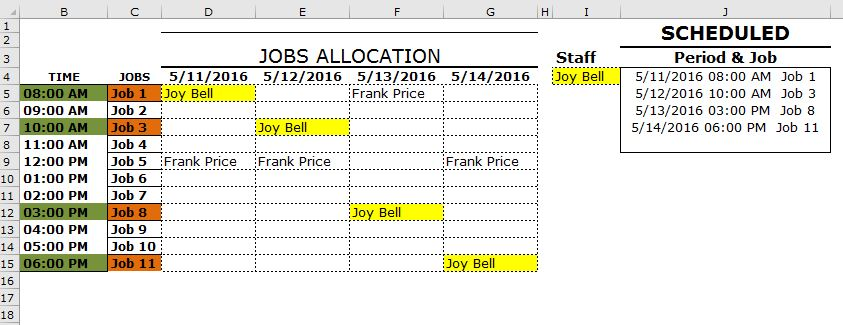#### Email Us

Follows

This is the last article in the series of reverse lookup in excel. Unless you are already versed with Reverse Lookup, consider checking below 2 articles first;

Now using VBA we are going to look up the date, time and Jobs assigned to Joy BellGo to Developer→Visual BAsic→Insert Module and copy below code

Now you can call the custom function on the worksheet.

```Function ReverseLookup(Staff As Range, Lookuptable As Range)

DatesRow = Lookuptable.Rows(1).Row - 1
TimeColumn = Lookuptable.Columns(1).Column - 2
JobColumn = Lookuptable.Columns(1).Column - 1

ReverseLookup = ""

For Each cell In Lookuptable

If cell.Value = Staff.Value Then

ReverseLookup = ReverseLookup & Cells(DatesRow, cell.Column).Value
& " " & Cells(cell.Row, TimeColumn).Value
& " " & Cells(cell.Row, JobColumn).Value & Chr(10)

End If

Next cell
End Function```

## Here is the Breakdown:

### ►Create a Function and pass two parameters

`Function ReverseLookup(Staff As Range, Lookuptable As Range)`
1.  What to lookup (Staff As Range)
2. Where to Lookup (Lookuptable As Range)

### ►Declare what you want to return:

• Dates which are  1 row above the lookup table ( DatesRow = Lookuptable.Rows(1).Row – 1   )
• Times which are  2  columns from the lookup table (TimeColumn = Lookuptable.Columns(1).Column – 2)
• Jobs which are  1  columns from the lookup table (JobColumn = Lookuptable.Columns(1).Column – 1)
NB: The Time Value have to be formatted as text first

### ►Declare a variable that will hold your results.

`ReverseLookup = ""`

NB. The variable should be in the same Name as your Function

### ►Declare a variable that the function will loop through

```For Each cell In Lookuptable

If cell.Value = Staff.Value Then```

For every cell that meets the above criteria, return the Date, Time and  Job & separate the result(s) with a carriage return (&Chr(10))

```ReverseLookup = ReverseLookup & Cells(DatesRow, cell.Column).Value
& " " & Cells(cell.Row, TimeColumn).Value
& " " & Cells(cell.Row, JobColumn).Value & Chr(10)```

## Recommended Videos

Mr Excel & excelisfun Trick 7: Reverse Lookup VBA or Formula?